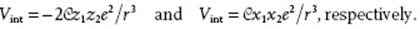﻿ ﻿Dispersion Interaction - Basic Energetics: Intermolecular Interactions - Liquid-State Physical Chemistry: Fundamentals, Modeling, and Applications (2013)

## Liquid-State Physical Chemistry: Fundamentals, Modeling, and Applications (2013)

### 3.4. Dispersion Interaction

Although the motion of electrons in a molecule is rather fast, at any moment the charge distribution of a molecule will have a (nonpermanent) dipole moment. The field associated with this dipole moment induces a (also nonpermanent) dipole moment in a neighboring molecule. Both induced dipoles interact, and this leads to the so-called (London) dispersion interaction, which is relatively weak but omnipresent and (in vacuum) always attractive. The exact derivation is outside the scope of these notes. However, a relatively simple model, proposed by London 7) and using the Drude model for atoms, yields good insight in the nature of the interaction. Moreover, it yields the correct expression apart from the exact numerical pre-factor.

In the Drude model, one assumes that an atom or molecule can be considered as a set of particles (electrons) with charge ei and mass mi. Each of these particles is harmonically and isotropically bound to its equilibrium position. The configuration and notation as used for a single electron is sketched in Figure 3.4. By considering the in-line configuration first, the dynamics of this electron are thus given by those of a harmonic oscillator for which we haveFigure 3.4 Schematic of the momentary interaction between the electrons (o) bonded to the nucleus (•) of two Drude atoms for an in-line orientation (a) and a parallel orientation (b).From quantum mechanics (see Section 2.2) we know that the energy expression becomeswhere n denotes the quantum number n = 0, 1, 2, 3, … and ω0 the circular frequency of the oscillator. The latter is given bywhere a is the force constant with which the electron is bound to the nucleus and m the electron mass. Without any interaction the total energy is given byIn the ground state, n = 0, the energy isAt any moment electron 1 of atom 1 exerts a force on electron 2 of atom 2 and for a distance r, using Eq. (3.8), the potential is given by

(3.13)so that the resulting total energy U isThis equation can be reduced to a sum of two independent harmonic oscillators, but with different frequencies. This is accomplished by the transformationHence, the solution becomeswith the new frequenciesand force constantsThe new ground-state energy becomesExpanding the square roots by the binomial theorem up to second order results in

(3.14)Finally, we link the force constant a to the polarizability α using the force eE of the electric field E as compensated by the restoring force az, that is, z = eE/a. The induced dipole moment is given by μ = ez = e2E/a as well as μ = αE, so that α = e2/a. The final expression for the in-line interaction energy becomes

(3.15)The dispersion energy is thus proportional to the polarizability α squared, a characteristic energy Iħω0 and the reciprocal sixth power of the distance r.

For the two parallel vibrations, perpendicular to the joining axis, of which the solutions are degenerated, a similar calculation leads to one-quarter of the above mentioned expression for each orientation, so that the total interaction becomes

(3.16)For dissimilar molecules, a similar but more complex calculation leads to

(3.17)This result is thus exact for the Drude model of a molecule with only one characteristic frequency, apart from the “binomial approximation”. For real molecules, the energies I should be chosen in accordance with the strongest absorption frequencies. In the absence of this information for I the ionization energies I0 are often taken. This seems to work reasonably well for He (I/I0 ≅ 1.20) and H2 (I/I0 ≅ 1.09). For the noble gases Ne, Ar, Kr and Xe, however, I/I0 should be taken about 9/4 in order to match more reliable calculations . A similar factor or even higher was noted for N2, Cl2, and CH4. In general, although estimates for α are fairly reliable (see Chapter 10), estimates for I are less trustworthy.

Problem 3.7

Show that Vint for the in-line configuration and the parallel orientation using the Drude model and the expressions for the dipole interactions is given byProblem 3.8

Verify for interacting oscillators the expression for U0′ and Win-line.

Problem 3.9*

Verify for interacting oscillators the expression for Wparallel.

Problem 3.10*

Derive the London dispersion interaction between dissimilar molecules.

﻿